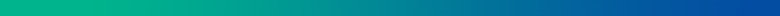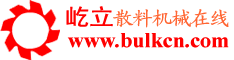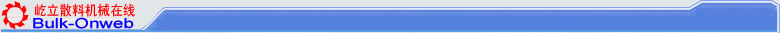您的位置：首页->技术论文->正文

DETERMINATION OF TRAVELLING SPEEDS OF BOTH  BELT STACKER AND  BOOM BELT

By Li Yimin

2001-3-5 By Li yimin    INSUE

In recent year, blending stackers are widely used in raw material yard of metallurgical enterprises as an equipment to blending stacking material. When blending stacker works the equipment travels to and fro along the rail of the train from the beginning to end. At the same time the equipment send the material sent by ground conveyor to boom conveyor and to yard . According to different stacking workmanship , stacking material may be divided in to two ways, ie.herringbone stacking way and rhombus stacking way , etc. .In order to make the volume of the stacked material equal, we must reasonably determine the velocity that the train forwards and backward ,so that the material could be  stacked evenly and reach a better result.
1.INSTANTANEOUS STACKING CAPACITY
Stacking capacity when the train stop :
Qt=60ArV   t/h (1)
Stacking capacity when the train goes forwards :
QQ=60Ar(Vo-V1)    t/h (2)
Stacking capacity when the train goes backward :
Qb=60Ar(V0+V2)    t/h  (3)
In the formula :

A--section area of the material on the belt of ground conveyor;

r--velocity of ground conveyor ,t/m3
V1--velocity when the train of the stacker goes forward , m/min;
V2--velocity when the train of the stacker goes backward , m/min;
when the direction that the train of the stacker and the direction of the belt of the ground conveyor are same ,we think it is advancing direction ,others therwise ,we think it is the backward direction.

2. DETERMINATION OF TRAVELLING SPEED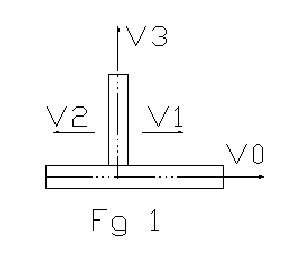In the course of blending stacker works we should make the stacked material volume on the travelling distance of the train equal when the blending stacker goes forward and goes backward .In such situation the thickness of nearby layers of material stacked on section of material pile can be nearly same ,we could get good blending result .That means that the material volume stacked when the equipment goes forwards and the material volume stacked when the equipment goes backwards is same.

Suppose: stacking material is q1 in a yield length of a material yard ,when the equipment goes forward.

so Qh=Ar(V0-V1)/v1                   t/h             (4)
suppose : stacking material is q2 in a yield length of a material yard ,when the equipment goes backwards  .
so Qh=Ar(V0+V2)/V2                    t/h           (5)
Order Qq=Qh
So

Ar(Vo-V1)/V1=Ar(Vo+V2)/V2                       (6)

The relation between belt velocity of ground conveyor and the travelling velocity of train is : (Vo-V1)/V1=(Vo+V2)/V2 (7)

There are two characteristics about determination of travelling velocity according to (7):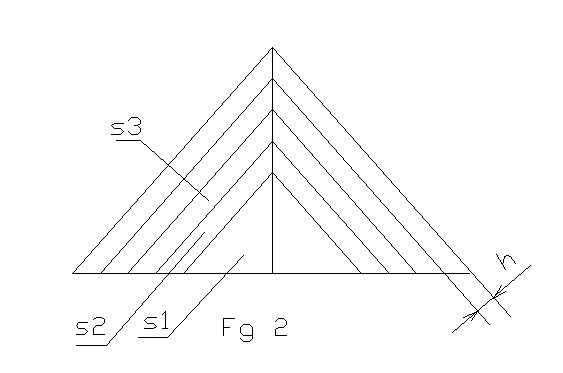1) Any two one-way travelling ,no matter it is going forward or going backwards, the material stacked on the yard is the same when the travelling distance of the train is same.  2)Section of material pile of any one-way on stacking section .Please Drawing 2.Suppose area is S, the thickness of material layer is h ,it will be s1=s2=s3=s4...si ;  When stacking layer reaches a certain value ,hn0 to hn+1

3.DETERMINATION OF BELT VELOCITY OF BOOM CONVEYOR
Suppose: the capacity of ground conveyor is Qt when blending stacking job is done ,the capacity of boom conveyor of when stacking is Qb ,so when the train stop the capacity of stacking boom conveyor is:

Qb=Qt=60ArV0                         t/h (9)

When the train forwards the capacity of the boom conveyor is:

Qb=Qq=60Ar(V0-V1)                 (10)

When the train backwards the capacity of the boom conveyor is:

Qb=Qh=60Ar(V0+V2)                     (11)

Among them Qh>Qt>Qq              (12)

So the belt velocity of stacking boom should be determined according to the capacity when the backwards . The conveyor capacity of stacking
Boom should be determined by following formula:

Qb=60A1rV3      t/h   (13)

In the formula ,A1 ----- section area of the material on the stacking
Conveyor ,m
V3 ------- belt velocity of stacking conveyor ,m/min
If Qb=Qh                t/h   (14)
So 60A1rV3=60Ar(V0+V2
A1V3=A(V0+V2
V3=A/A1(V0+V2)      m/min   (15)
If the Belt width of stacking boom conveyor and belt width of ground
Conveyor is same , and incling degree is nearly same ,under such condition ,
the belt of boom conveyor should be determined by following formula :
V3=V0+V2 m/min (16)

FROM <SINTERING AND PELLETIZING >  ISSN1000-8764 1992-3

2001-3-5 By Li yimin    INSUE

《屹立散料机械在线》《散料机械技术》